### ESM-RISM

In :
%load_ext autoreload
%matplotlib inline
from pyscript import PlotQE_RISM as pt
from IPython.display import SVG, display, Image
import numpy as np
import matplotlib.pyplot as plt
import math


# Tutorial for DFT with reference interaction site model (RISM) calculation¶

This tutrial is aiming to utilize the density functional theory (DFT) with reference interaction site model (RISM) calculation implemented in the QUANTUM ESPRESSO code.

The DFT calculation treats the electronic structures within quantum mechanical theory. On the other hand, the RISM is a classical solution theory and solution is treated using classical force field.
Thus, the DFT+RISM can calculate the electronic structure of the atoms, ions, molecules, materials in the solution. In this framework, solvent charge densities are treated as implicit solvent with fixed inner moleculer structures.
Moreover, the great advantage of the DFT+RISM is that the electrode/electrolyte interface can be precisely treated under the grand canonical condition for solute ions and molecules.

Theoretical back-ground is briefly described in S. Nishihara and M. Otani, Phys. Rev. B 96, 115429 (2017). Please see the paper first of all, because understanding the theoretical consept is very helpful for learning the RISM calculation.

Three types of RISM calculation method is implemented in PWscf code.

This tutorial is organized as follows:

1. Input values and files for RISM calculation
2. 3D-RISM calculation for H$_2$O molecule in the NaCl aq.
3. ESM-RISM calculation for Al(111) slab in the NaCl aq.
4. Determining Standard Hydrogen Electrode potential using ESM-RISM calculation

## 0. Input values for RISM calculation¶

Before starting the DFT+RISM calculation, we show the input values and files for the RISM calculation.
Following lists and disctiptions should be refered, when you run the RISM calculation.

1. Input values for RISM calculation
2. *.MOL files

### 0-1. Input values for RISM calculation¶

Here, commonly used input values for the RISM calculation are briefly described.

### Namelist: &CONTROL¶

##### trism: LOGICAL/default = .FALSE.¶

This is a swich for the RISM calculation. If this vaule is not setted, RISM related input values will be neglected. If this value is activated, the &RISM namelist and SOLVENS card is required. In the RISM calculation, the distribution of solvent is calculated by the RISM theory, and the explicit solute (such as surface slab) is treated by the DFT.
If assume_isolated = esm and esm_bc=bc1 is used, the Laue-RISM calculation will be carried out.

The default of mixing_beta is 0.2 for both 3D-RISM and Laue-RISM.

For structural relaxation with BFGS, ignore_wolfe is always .TRUE. .

### Namelist: &RISM¶

This namelist describes the main input-values for RISM calculation. This namelist can be utlized with trism=.TRUE..

##### nsolv: INTEGER/default = 0¶

The number of solvents (i.e. molecular species) in the unit cell. This value must be required in the RISM calculation.

##### closure: CHARACTER/default = 'kh'¶

Specify the type of closure equation.
Now, we can use two types of closure equations:

• 'kh': the Kovalenko and Hirata model [A.Kovalenko, F.Hirata, JCP 110, 10095 (1999)]
• 'hcn': HyperNetted-Chain model [J.P.Hansen et al., Theory of simple liquids. Academic Press, London, 1990]

NOTE: 'kh' closure is highly recommended.

##### tempv: REAL/default = 300.0¶
Temperature of solvents are determind (units in Kelvin).



##### ecutsolv: REAL/default = 4 $\times$ ecutwfc¶

Kinetic energy cutoff (unit in Ry) for the correlation functions of solvent. If a solute is an isolated system or slab, you may allowed to use default value. or a frameworked or porous solute (e.g. Zeolite, MOF), it is desirable to apply a larger value. Solvents confined in a framework often have a high frequency.

##### solute_lj(1:ntyp): CHARACTER/default = 'uff'¶

Specify the Lennard-Jones potential type of solute-solvent interactions for itypth solute atomic type:

• 'uff': Universal Force Field [A.K.Rappe et al., JACS 144, 10024 (1992)]
• 'clayff': Clay's Force Field [R.T.Cygan et al., JPC B 108, 1255 (2004)]
• 'opls-aa': OPLS-AA (generic parameters for QM/MM) [see https://github.com/thatchristoph/vmd-cvs-github/blob/master/plugins/bossconvert/oplsaa.par]
• 'none': The Lennard-Jone's parameters are specified by user with solute_epsilon and solute_sigma.

NOTE: This value is utilized in the 3D-RISM or Laue-RISM calculation.

##### solute_epsilon(1:ntyp): REAL/default =¶

Specify the Lennard-Jones parameter $\varepsilon$ (unit in kcal/mol) for itypth atomic type.
This value must set in the case of solute_lj(1:ntyp)='none'.

##### solute_epsilon(1:ntyp): REAL/default =¶

Specify the Lennard-Jones parameter $\sigma$ (unit in Å) for itypth atomic type.
This value must set in the case of solute_lj(1:ntyp)='none'.

##### starting1d: CHARACTER/default = 'zero'¶

Starting point for correlation functions obtained by the 1D-RISM calculation.

• 'zero': Starting correlation functions are newly calculated by the 1D-RISM.
• 'file': Starting correlation functions are read from the existing 1d-rism_csvv_r.xml file.
In the restart calculation mode, this value is the default.
• 'fix': Starting correlation functions are read from existing "1d-rism_csvv_r.xml" file,
and never calculate 1D-RISM within nscf and bands calculation.

##### starting3d: CHARACTER/default = 'zero'¶

Starting point for correlation functions obtained by the 3D-RISM or Laue-RISM calculation.

• 'zero': Starting correlation functions are newly calculated by the 3D-RISM.
• 'file': Starting correlation functions are read from the existing 1d-rism_csvv_r.xml file.
In the restart, nscf and bands calculation mode, this value is the default.

##### smear1d: REAL/default = 2.0¶

Coulomb smearing radious for the 1D-RISM.
NOTE: Usually, this value is not changed.

##### smear3d: REAL/default = 2.0¶

Coulomb smearing radious for the 3D-RISM and Laue-RISM.
NOTE: Usually, this value is not changed.

##### rism1d_maxstep: INTEGER/default = 5000¶

Maximum number of iterations in 1D-RISM cycle.
NOTE: rism1d_maxstep=10000 or more than 10000 is recommended.

##### rism3d_maxstep: INTEGER/default = 5000¶

Maximum number of iterations in the 3D-RISM and Laue-RISM cycle.
NOTE: rism1d_maxstep=10000 or more than 10000 is recommended.

##### rism1d_conv_thr: REAL/default = 1.d-8¶

Convergence criteria for the 1D-RISM.
NOTE: the default value is recommended.

##### rism3d_conv_thr: REAL/default = 1.d-5¶

Convergence criteria for the 3D-RISM and Laue-RISM.
NOTE: rism3d_conv_thr=1.d-6 is recommended, because we found that the Fermi level depends on the convergence criteria.

##### mdiis1d_size INTEGER/default = 20¶

Size of Modified DIIS (MDIIS) for the 1D-RISM.
MDIIS is one of the robust optimization method to obtain converged correlation functions.

##### mdiis3d_size INTEGER/default = 10¶

Size of Modified DIIS (MDIIS) for the 3D-RISM and Laue-RISM.
NOTE: mdiis3d_size $\geq$ 10 is recommended.

##### mdiis1d_step REAL/default = 0.5¶

Step of MDIIS for the 1D-RISM.
NOTE: mdiis1d_step $=$ 0.1 is recommended.
If your 1D-RISM calculation cannot obtain the convergence more than 10000 steps,
you try to decrease mdiis1d_step from 0.1 or increase from 0.1 to 0.12.

##### mdiis3d_step REAL/default = 0.8¶

Step of MDIIS for the 3D-RISM and Laue-RISM.
NOTE: If your 3D- or Laue-RISM calculation cannot obtain the convergence,
you try to change mdiis3d_step.

##### rism1d_bond_width REAL/default =¶

Gaussian width of bonds to smear intra-molecular correlation for 1D-RISM.

• 3D-RISM: default = 0.0
• Laue-RISM: default = $2/\sqrt{\rm ecutwfc}$.

##### rism1d_nproc INTEGER/default = 128¶

Number of processes for calculating 1D-RISM.
If you use the number of processes for FFT grids $\leq 128$, rism1d_nproc is automatically determined with sutable value.

##### rism3d_conv_level REAL/default = 0.1¶

Convergence level of the 3D-RISM.
Default value of rism3d_conv_level is set to 0.3 with lgcscf=.TRUE..

rism3d_conv_level must be set in the range of [0.0, 1.0].

• rism3d_conv_level = 0.0: Convergence level is 'low'.
Convergence criteria for the 3D- or Laue-RISM is greater than rism3d_conv_thr, when estimated energy error >> conv_thr.
• 0.0 < rism3d_conv_level < 1.0: Convergence level is 'medium'.
Convergence criteria for the 3D- or Laue-RISM is intermediate value between 'low' and 'high', where rism3d_conv_level is mixing rate.
• rism3d_conv_level = 1.0: Convergence level is 'high'.
Convergence threshold of 3D- or Laue-RISM always equals to rism3d_conv_thr.

NOTE: Iterative method for 3D- or Laue-RISM highly depends on the solution system. Sometimes, your RISM-SCF calculation might not be achieved convergence. In such case, rism3d_conv_level is very useful for achieving the convergence.
According to our experience, rism3d_conv_level = 0.5 or 1.0 is better value for 3D- or Laue-RISM.

##### rism3d_planar_average LOGICAL/default =¶

Controlling output level for laterally averaged solvent and solute charge densities and electrostatic potentials.
In the Laue-RISM calculation, rism3d_planar_average is .TRUE. and above quantities are written in outdir/prefix.rism1 file.

##### laue_nfit INTEGER/default = 4¶

The number of z-grid points for the polynomial fit along the cell edge like the ESM calculation. This is only used for the Laue-RISM.

##### laue_expand_right REAL/default = -1.0¶

This is only used for the Laue-RISM. The positive value of laue_expand_right sets the ending position offset (unit in bohr) of the solvent region on right-hand side of the unit cell, measured relative to the unit cell edge (the solvent region ends at z = + [L_z/2 + laue_expand_right].) . Here, L_z is unit cell length for z-direction.

##### laue_expand_left REAL/default = -1.0¶

This is only used for the Laue-RISM. The positive value of laue_expand_left sets the ending position offset (unit in bohr) of the solvent region on left-hand side of the unit cell, measured relative to the unit cell edge (the solvent region ends at z = - [L_z/2 + laue_expand_left].) . Here, L_z is unit cell length for z-direction.

##### laue_starting_right REAL/default = 0.0¶

This is only used for the Laue-RISM. Set the starting position (unit in bohr) of the solvent region on right-hand side of the unit cell. Then the solvent region is defined as [ laue_starting_right , L_z/2 + laue_expand_right ], where distribution functions are finite. Here, L_z is unit cell length for z-direction.

##### laue_starting_left REAL/default = 0.0¶

This is only used for the Laue-RISM. Set the starting position (unit in bohr) of the solvent region on right-hand side of the unit cell. Then the solvent region is defined as [ -(L_z/2 + laue_expand_left), laue_starting_left ], where distribution functions are finite. Here, L_z is unit cell length for z-direction.

##### laue_buffer_right REAL/default =¶

This is only used for the Laue-RISM.
The default value is set as following:

• laue_buffer_right = 8.0, if laue_expand_right > 0.0
• laue_buffer_right = -1.0, if laue_expand_right $\leq$ 0.0

If positive value, set the buffering length (unit in bohr) of the solvent region on right-hand side of the unit cell. Then correlation functions are defined inside of [laue_starting_right-laue_buffer_right, L_z/2 + laue_expand_right ]. Here, L_z is unit cell length for z-direction.

##### laue_buffer_left REAL/default =¶

This is only used for the Laue-RISM.
The default value is set as following:

• laue_buffer_left = 8.0, if laue_expand_left > 0.0
• laue_buffer_left = -1.0, if laue_expand_left $\leq$ 0.0

If positive value, set the buffering length (unit in bohr) of the solvent region on left-hand side of the unit cell. Then correlation functions are defined inside of [-(L_z/2 + laue_expand_left), laue_starting_left+laue_buffer_left]. Here, L_z is unit cell length for z-direction.

### Card: SOLVENTS¶

Optional card, used for specifying the solvent molecules, solvent MOL files and solvent densities in the RISM calculation.
If trism is .FALSE., this card will be neglected.

#### Syntax:¶

SOLVENTS {Density_unit}
Molecule_name(1), Density(1), MOL_file(1)
....
Molecule_name(nsolv), Density(nsolv), MOL_file(nsolv)

#### Description of items:¶

##### Density_unit: CHARACTER¶
• 1/cell: solvent density is defined as the number of molecules in unit cell.
• mol/L: solvent density is defined as molar.
• g/cm^3: solvent density is defined in gram per cm$^3$.
##### Molecule_name: CHARACTER¶

Labels of the solvent molecules.

##### density: REAL¶

Densities of the solvent molecules (unit in Density_unit). If density is negative, the density of solvent molecule is read from specified MOL file.

##### MOL_file: CHARACTER¶

.MOL file of the solvent molecule. Descriptions for .MOL file is found to be next section.

### 0-2. MOL files¶

.MOL files include the density of moleculars and ions, charge of moleculers and ions, xyz-file type moleculer structures, Lennard-Johnes parameters for each atom and so on. To run the RISM calculations, .MOL files must be stored in pseudo_dir/, which is the path for pseudopotential files.

Necessary .MOL and pseudopotential files for this tutorials are given in ./MOL/ directry.
If you want to use new solute or solvent molecules and ions, please visit and get them at the Satomichi Nishihara's Github repogitory [https://github.com/nisihara1/MOLs]. If you do not find required MOL files, you try to make the new MOL files for your purpose.

## 1. 3D-RISM calculation for H$_2$O molecule in the NaCl aq.¶

In this section, we carry out the 3D-RISM calculation for explicit solute of H$_2$O molecule in implicit solvent of NaCl aq (1mol).
Following input file is used for this tutorial. First of all, please copy the input, necessary *.MOL and pseudopotential files to your calculation directry (.MOL and pseudopotential files are given in ./MOL/ and ./pseudo/ directries, respectively).

In this calculation, we use Lennard-Jones (LJ) parameters of spc model for water molecule.
The RISM calculation is a kind of model calculation, and we should always note the treatment of input parameters.
If you want to know LJ-parameters before calculation, please open your .MOL file and read them.

Next, open the input file using editor tool, and edit pseudo_dir path to your pseudopotential file path, and then, please read your input and understand your calculation. If you forgot the meanings of inputs, please check again the input list above.

After reading and understanding the input file, the preperation for 3D-RISM calculation is done. Let's run your first 3D-RISM calculation using pw.x.

&control
calculation  = 'relax'
prefix       = 'H2O.NaCl_aq.1mol'
pseudo_dir   = './pseudo'
outdir       = './output'
trism        = .true.
nstep        = 100
/
&system
ibrav = 1, a = 20.0
ntyp = 2, nat = 3
ecutwfc = 40.0, ecutrho = 320.0
nbnd = 8
occupations = 'smearing', smearing = 'gauss', degauss = 0.01
/
&electrons
electron_maxstep = 200
/
&ions
ion_dynamics = 'bfgs'
/
&rism
nsolv    = 3, closure  = 'kh'
tempv    = 300.0  ! Kelvin
ecutsolv = 160.0  ! Rydberg
!
! Lennard-Jones for each atom (SPC model)
!
! For O (TIP5P)
solute_lj(1) = 'none'
solute_epsilon(1) = 0.1554  ! kcal/mol
solute_sigma(  1) = 3.1660  ! angstrom
! For H (modified H)
solute_epsilon(2) = 0.0460
solute_sigma(  2) = 1.0000
!
! 1D-RISM's setting
!
starting1d      = 'zero'
rism1d_conv_thr = 1.0e-8
rism1d_maxstep  = 10000
mdiis1d_size    = 20
mdiis1d_step    = 0.5
!
! 3D-RISM's setting
!
starting3d      = 'zero'
rism3d_maxstep  = 10000
rism3d_conv_thr = 1.0e-6
/
ATOMIC_SPECIES
O  -1.0  O.pbe-n-rrkjus_psl.1.0.0.UPF
H  -1.0  H.pbe-rrkjus_psl.1.0.0.UPF
ATOMIC_POSITIONS {angstrom}
O  10.00000  10.00000  10.00000  0 0 0
H  10.81649  10.57736  10.00000
H   9.18351  10.57736  10.00000

K_POINTS {gamma}

SOLVENTS {mol/L}
H2O  -1.0  H2O.spc.MOL ! Now we use density of H2O
Na+   1.0  Na+.aq.MOL
Cl-   1.0  Cl-.aq.MOL

After running of the RISM calculation correctly, we can obtain the results of 1D- and 3D-RISM calculation.

First, please open your calculation log file, and you can find results of chemical potential of solvetion determined by the 1D-RISM.
Total values for each molecule and ion corresponds to the solvation free energy.

Second, you can find the 3D-RISM calculation log in the log file.
Here, you can also find chemical potential of solvation, total number and charge of solvent and total energy of DFT+RISM calculation etc...

In this calculation, structure optimization for explicit water molecule is also carried out. Please check your forces acting on atoms, which will be decrease with reaching convergence of whole calculation.

Next, we check the further 1D-RISM calculation results. Below /WORKDIR/output/ directry, H2O.NaCl_aq.1mol.1drism file might be created after calculation.
This file contains the pair-distribution function data for atomic pairs consisted with the solution.
Below, we visualize all pair-distribution functions. It is noted that this distribution functions are normalized to one in the limit of huge $r$. Thus, densities for each molecule are not multiplied.

In :
pt.plot_1d_rism( path = './data/H2O.NaCl_aq',
prefix = 'H2O.NaCl_aq.1mol', normalization = True, limx = 10.0 )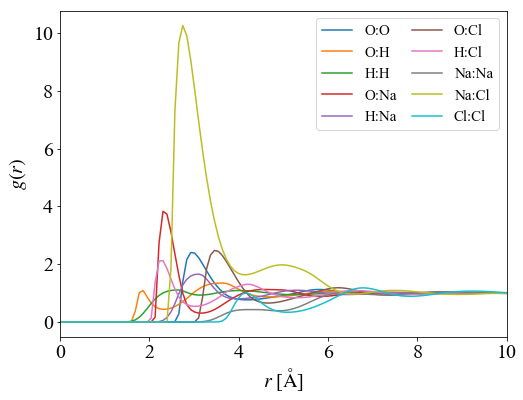We calculate the number of atoms around target atoms using results of the 1D-RISM as for example.
The number of atoms can be evaluated by the solute densities and distribution functions as following:

\begin{align} N_{\rm at}= \int 4\pi r^2 \rho g(r) dr \end{align}

Below, we plot the number of atoms as well as radial distribution functions. The blue and red curves denote the distribution function for O-Na$^+$ and Na$^+$-Cl$^-$ pairs, respectively. Orange and green curves respectively indicate the number of O and Cl$^-$ atoms around Na$^+$ ions. A single O atom and Cl$^-$ ion is respectively found at the first-peak positions of distribution functions.

In :
pt.sum_1drism()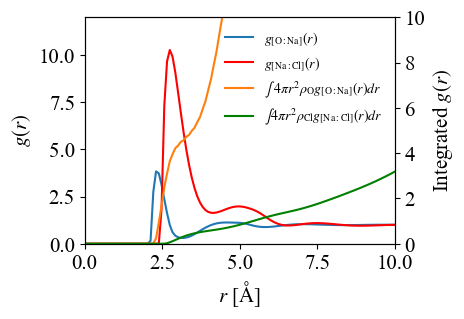For further analysis, we also visualize the charge distribution of solvent for the 3D-RISM.
To make 3D-density distribution file, we use pprism.x execution file.
pprism.x requires an input file whose format is similar to that for pp.x. Input file for pprism.x is shown below.

&inputpp
outdir  = './output'
prefix  = 'H2O.NaCl_aq.1mol'
lpunch  = .true.
/
&plot
output_format = 6
iflag = 3
nx = 160, ny = 160, nz = 160
x0(1) = 0.0
x0(2) = 0.0
x0(3) = 0.0
interpolation = 'fourier'
/

After running pprism.x, we obtain following cube file (output_format=6):

• H2O.NaCl_aq.1mol.3drism_chg.cube
• H2O.NaCl_aq.1mol.3drism_rho#1_O.cube
• H2O.NaCl_aq.1mol.3drism_rho#2_H.cube
• H2O.NaCl_aq.1mol.3drism_rho#3_Na.cube
• H2O.NaCl_aq.1mol.3drism_rho#4_Cl.cube
• H2O.NaCl_aq.1mol.3drism_v_solu.cube
• H2O.NaCl_aq.1mol.3drism_v_solv.cube
• H2O.NaCl_aq.1mol.3drism_v_tot.cube

Here, we plot the H2O.NaCl_aq.1mol.3drism_chg.cube using VESTA package.

In :
SVG(filename='./FIGs/H2O_NaCl_aq_3D_RISM.svg')

Out:

Contour map for charge distribution of solvent around explicit H$_2$O molecule.

Since the O atom in H$_2$O molecule is negatively charged, positive charge of solvent (red) is attracted by O atom due to the Coulomb interaction.
On the other hand, the H atoms is positively charged. Because of this, negative charge of solvent (blue) is distribute near H atoms.
Furthermore, the charge distribution becomes neutral (green) at the region far from the solute. In this region, we can understand that the Coulomb interaction is screened by the solvent.

As discussed above, visualizing the charge distribution of solvent around explicit solute is very helpful for understanding the solvent-solute interactions.

## 2. ESM-RISM calculation for Al(111) slab in the NaCl aq.¶

Here, we run five calculations as following:

• 2-1. ESM-RISM calculation for Al(111) slab with neutral charge in the NaCl aq.
• 2-2. ESM-RISM calculation for Al(111) slab with positive charge in the NaCl aq.
• 2-3. ESM-RISM calculation for Al(111) slab with negative charge in the NaCl aq.
• 2-4. ESM-RISM calculation for Al(111) slab with explicit solvent ion (Na$^+$) in the NaCl aq.
• 2-5. ESM-RISM calculation for Al(111) slab with explicit solvent ion (Cl$^-$) in the NaCl aq.

In these calculations, the ESM-RISM calculations are carried out.
In the ESM-RISM, assume_isolated = "esm" and esm_bc = "bc1" is necessary for using the open-boundary condition.
Thus, we use periodic- and open-boundary conditions for latellal and surface normal directions, respectively.

Here, we briefly describe the advantage of the ESM-RISM calculation. The ESM-RISM framework can treat charged surface because of grand-canonical conditions. Ordinarily DFT framework with periodic boundary condition cannot treat charged surface because the electrostatic potential terms are diverged. To avoid the divergence, jellium backgraund is usually introduced. However, the origin of total energy cannot be uniquely defined. For this reason, treatment of interface between charged surface and solution was difficult.

However, in the ESM-RISM, the charge neutral condition is always satisfied with whole system that is consisted of the solute and solvent.
For this reason, the ESM-RISM is a very useful tool to study on the material/solution interface such as the electrochemical interface.

### 2-1. ESM-RISM calculation for Al(111) slab with neutral charge in the NaCl aq.¶

First, we learn how to run the ESM-RISM calculation using Al(111)/NaCl aq. interface.
Let's read input file and values for understanding the ESM-RISM calculation. In &system, you can find the description of the ESM calcuation with bc1 (Vacuum/Slab/Vacuum). Thus, we use mixed boundary condition as open boundary condition for z-direction and periodic boundary condition for xy-plane. It is note that the definition of ATOMIC_POSITIONS is as same as ordinary ESM calcuation in the ESM-RISM calculation (Thus, origin of unit cell is shift to the center of unit cell. ).
In &rism, we find that the new input laue_expand_right. This value determine the expand unit cell length for the ESM-RISM. In the ESM-RISM, the solute density distribution far from the surface should be constant. Thus, enough thickness of the vacuum layer. However, DFT calculation with the large vacuum layer requires huge computational costs. To avoid huge calculation, we use expand cell techuniqe with laue_expand_right(or laue_expand_left). Details for laue_expand_right can be found in the input list of the RISM calculation.

Please, change the pseudopotential file path (pseudo_dir) as sutable for your enviroment and execute pw.x.

&control
calculation  = 'scf'
prefix       = 'Al111.NaCl_aq'
pseudo_dir   = './pseudo'
outdir       = './output'
trism        = .true.
/
&system
ibrav = 0
ntyp = 1, nat = 3
ecutwfc = 25.0, ecutrho = 200.0
nbnd = 8
occupations = 'smearing', smearing = 'gauss', degauss = 0.01
assume_isolated = "esm", esm_bc = "bc1", tot_charge = 0.0
/
&electrons
electron_maxstep = 200
mixing_beta = 0.1
diagonalization = 'rmm', diago_rmm_conv = .false., diago_rmm_ndim = 4
/
&rism
nsolv    = 3, closure  = 'kh'
tempv    = 300.0  ! Kelvin
ecutsolv = 160.0  ! Rydberg
!
! Lennard-Jones for each atom
!
! For Al using universal force field
solute_lj(1) = 'uff'
!
! 1D-RISM's setting
!
starting1d      = 'zero'
rism1d_conv_thr = 1.0e-8
rism1d_maxstep  = 10000
mdiis1d_size    = 20
mdiis1d_step    = 0.5
!
! 3D-RISM's setting
!
starting3d      = 'zero'
rism3d_maxstep  = 10000
rism3d_conv_thr = 1.0e-6
!
! For Laue-RISM calculation
! in a.u.
!
laue_expand_right = 100.0
/
ATOMIC_SPECIES
Al  -1.0  Al.pbe-nl-rrkjus_psl.1.0.0.UPF
CELL_PARAMETERS angstrom
2.85671139599365 0.00000000000000 0.00000000000000
1.42835569799683 2.47398464021101 0.00000000000000
0.00000000000000 0.00000000000000 35.0000000000000
ATOMIC_POSITIONS angstrom
Al 1.4283556980 0.8246615467 -2.3324950875
Al 0.0000000000 1.6493230935 -0.0000000000
Al 0.0000000000 0.0000000000  2.3324950875
K_POINTS {automatic}
8 8 1 1 1 0
SOLVENTS {mol/L}
H2O  -1.0  H2O.spc.MOL
Na+   1.0  Na+.aq.MOL
Cl-   1.0  Cl-.aq.MOL

After finishing the ESM-RISM calculation, prefix.rism1 files are created below output/ directry. This file contains important results of the ESM-RISM calculations. prefix.rism1 file contains information on laterally averaged electrostatic potentials and charge densities of solution. Below, we plots the results written in prefix.rism1 files.

Below, we plot the electrostatic potentials of solution (left) and charge densities of the NaCl aq. solvent (center and right).

###### Electrostatic potentials¶

Solvent potential (black line) shows oscilation near the Al surface, because of surface-solvent interaction via the sum of Lennard-Jones and electrostatic potentials. This oscilation can be also found in total potential (blue line).
In the open boundary condition, energy-zero level is defined by left and right vacuum level. However, in the ESM-RISM, we shift the origin of energy to the solvent bulk region.

###### Solvent densities¶

Solvent densities distribute from about 4.5Å to $z\rightarrow \infty$ measured from the slab center. Furthermore, solvent densities show the oscilations near the surface to about 20Å, which converge to the constant. These oscilations are caused by interaction between the surface and solvent via the sum of LJ and electrostatic potentials. The constant density-distributions indicate uniform distribution of solute.
In addition to above, uniform distribution of solute density allow us to calculate grand-canonical condition. Thus, uniform density plays a role of reserver of solute. This is an important advantage of the ESM-RISM calculation. Following charged surface calculations show this advantage.

In :
pt.plot_rism_NaCl_aq1( path = './data/Al111.NaCl_aq',
prefix = 'Al111.NaCl_aq' )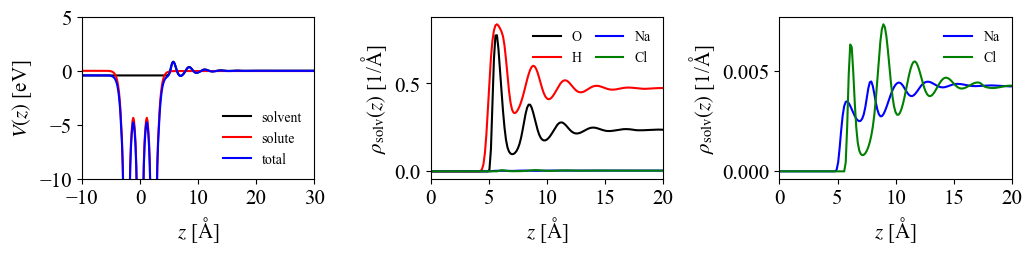### 2-2. ESM-RISM calculation for Al(111) slab with positive charge in the NaCl aq.¶

Second, we calculate an interface between Al(111) surface with positive charge and NaCl aq.. In this calculation, surface charge $\sigma=+0.02e$ is assumed using tot_charge. Other inputs are as same as Sec. 2-1..

&control
calculation  = 'scf'
prefix       = 'Al111-0.02e.NaCl_aq'
pseudo_dir   = './pseudo'
outdir       = './output'
trism        = .true.
/
&system
!   ibrav = 1, a = 10.0
ibrav = 0
ntyp = 1, nat = 3
ecutwfc = 25.0, ecutrho = 200.0
nbnd = 8
occupations = 'smearing', smearing = 'gauss', degauss = 0.01
assume_isolated = "esm", esm_bc = "bc1", tot_charge = 0.02
/
&electrons
electron_maxstep = 200
mixing_beta = 0.1
diagonalization = 'rmm', diago_rmm_conv = .false., diago_rmm_ndim = 4
/
&rism
nsolv    = 3, closure  = 'kh'
tempv    = 300.0  ! Kelvin
ecutsolv = 160.0  ! Rydberg
!
! Lennard-Jones for each atom
!
! For Al using universal force field
solute_lj(1) = 'uff'
!
! 1D-RISM's setting
!
starting1d      = 'zero'
rism1d_conv_thr = 1.0e-8
rism1d_maxstep  = 10000
mdiis1d_size    = 20
mdiis1d_step    = 0.5
!
! 3D-RISM's setting
!
starting3d      = 'zero'
rism3d_maxstep  = 10000
rism3d_conv_thr = 1.0e-6
!
! For Laue-RISM calculation
! in a.u.
!
laue_expand_right = 100.0
/
ATOMIC_SPECIES
Al  -1.0  Al.pbe-nl-rrkjus_psl.1.0.0.UPF
CELL_PARAMETERS angstrom
2.85671139599365 0.00000000000000 0.00000000000000
1.42835569799683 2.47398464021101 0.00000000000000
0.00000000000000 0.00000000000000 35.0000000000000
ATOMIC_POSITIONS angstrom
Al 1.4283556980 0.8246615467 -2.3324950875
Al 0.0000000000 1.6493230935 -0.0000000000
Al 0.0000000000 0.0000000000  2.3324950875
K_POINTS {automatic}
8 8 1 1 1 0
SOLVENTS {mol/L}
H2O  -1.0  H2O.spc.MOL
Na+   1.0  Na+.aq.MOL
Cl-   1.0  Cl-.aq.MOL

After running the ESM-RISM, results of electrostatic potentials and charge densities of solute are plotted.
The electrostatic potentials for solute and solvent show the slope structure in the vacuum and solvent regions, which is shown in the right panel. However, the total potential shows flat structure in the the vacuum and solvent regions. This result indicates that the surface charge is completely screened by the induced counter charge in the ions of electrolyte. In the right panel, the charge density of Cl$^-$ near the surface is increased compareing to that at the charge neutoral surface. On the other hand, the change in the charge density of Na$^+$ shows opposite tendency to that of Cl$^-$. The change in the charge density distributions are caused by the Coulomb interaction between the induced surface charge and solute.

In :
pt.plot_rism_NaCl_aq1( path = './data/Al111.NaCl_aq',
prefix = 'Al111-0.02e.NaCl_aq' )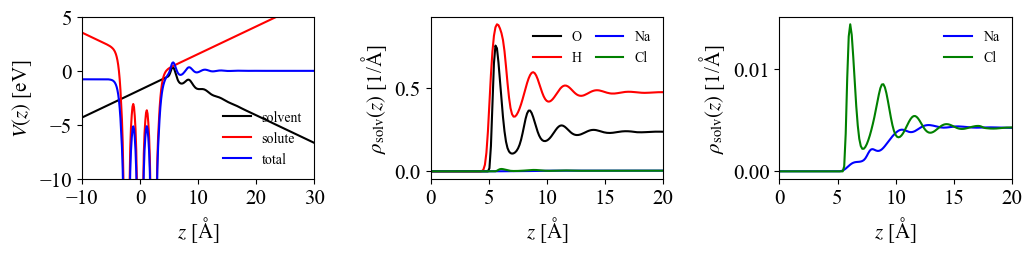### 2-3. ESM-RISM calculation for Al(111) slab with negative charge in the NaCl aq.¶

Third, we calculate an interface between Al(111) surface with $\sigma=-0.02e$ and NaCl aq. for comparing to the $\sigma=-0.02e$ case.

&control
calculation  = 'scf'
prefix       = 'Al111+0.02e.NaCl_aq'
pseudo_dir   = './pseudo'
outdir       = './output'
trism        = .true.
/
&system
ibrav = 0
ntyp = 1, nat = 3
ecutwfc = 25.0, ecutrho = 200.0
nbnd = 16
occupations = 'smearing', smearing = 'gauss', degauss = 0.01
assume_isolated = "esm", esm_bc = "bc1", tot_charge = -0.02
/
&electrons
electron_maxstep = 200
mixing_beta = 0.1
diagonalization = 'rmm', diago_rmm_conv = .false., diago_rmm_ndim = 4
/
&rism
nsolv    = 3, closure  = 'kh'
tempv    = 300.0  ! Kelvin
ecutsolv = 160.0  ! Rydberg
!
! Lennard-Jones for each atom
!
! For Al using universal force field
solute_lj(1) = 'uff'
!
! 1D-RISM's setting
!
starting1d      = 'zero'
rism1d_conv_thr = 1.0e-8
rism1d_maxstep  = 10000
mdiis1d_size    = 20
mdiis1d_step    = 0.5
!
! 3D-RISM's setting
!
starting3d      = 'zero'
rism3d_maxstep  = 10000
rism3d_conv_thr = 1.0e-6
!
! For Laue-RISM calculation
! in a.u.
!
laue_expand_right = 100.0
/
ATOMIC_SPECIES
Al  -1.0  Al.pbe-nl-rrkjus_psl.1.0.0.UPF
CELL_PARAMETERS angstrom
2.85671139599365 0.00000000000000 0.00000000000000
1.42835569799683 2.47398464021101 0.00000000000000
0.00000000000000 0.00000000000000 35.0000000000000
ATOMIC_POSITIONS angstrom
Al 1.4283556980 0.8246615467 -2.3324950875
Al 0.0000000000 1.6493230935 -0.0000000000
Al 0.0000000000 0.0000000000  2.3324950875
K_POINTS {automatic}
8 8 1 1 1 0
SOLVENTS {mol/L}
H2O  -1.0  H2O.spc.MOL
Na+   1.0  Na+.aq.MOL
Cl-   1.0  Cl-.aq.MOL

### Comparison of potentials and densities between charged and neutral surfaces¶

In :
print('* Charge zero')
pt.plot_rism_NaCl_aq1( path = './data/Al111.NaCl_aq',
prefix = 'Al111.NaCl_aq' )
print('* Charge +0.02e')
pt.plot_rism_NaCl_aq1( path = './data/Al111.NaCl_aq',
prefix = 'Al111-0.02e.NaCl_aq' )
print('* Charge -0.02e')
pt.plot_rism_NaCl_aq1( path = './data/Al111.NaCl_aq',
prefix = 'Al111+0.02e.NaCl_aq' )

* Charge zero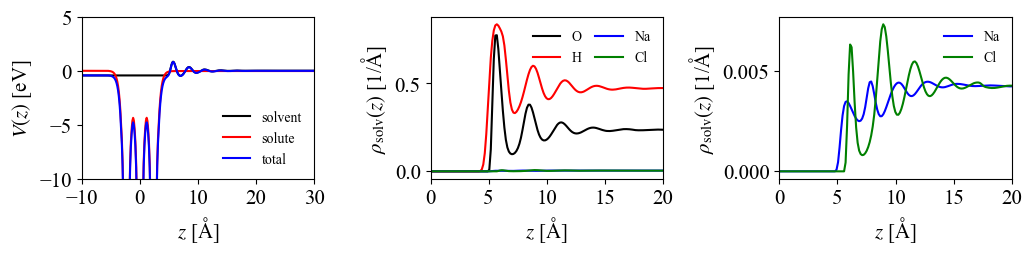* Charge +0.02e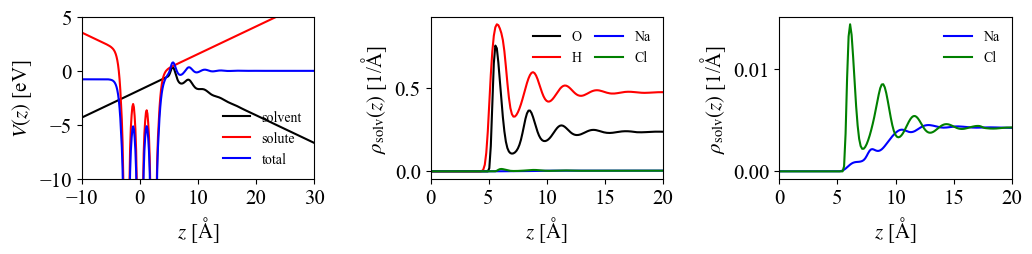* Charge -0.02e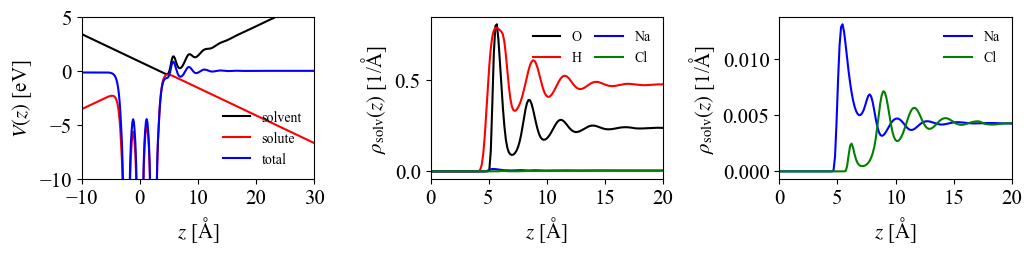### 2-4. ESM-RISM calculation for Al(111) slab with explicit solvent ion (Na$^+$) in the NaCl aq.¶

&control
calculation  = 'scf'
prefix       = 'Al111.NaCl_aq'
pseudo_dir   = './pseudo'
outdir       = './output'
trism        = .true.
/
&system
!   ibrav = 1, a = 10.0
ibrav = 0
ntyp = 2, nat = 28
ecutwfc = 40.0, ecutrho = 320.0
occupations = 'smearing', smearing = 'gauss', degauss = 0.01
assume_isolated = "esm", esm_bc = "bc1", tot_charge = 1.0
/
&electrons
electron_maxstep = 200
mixing_beta = 0.1
diagonalization = 'rmm', diago_rmm_conv = .false., diago_rmm_ndim = 4
/
&rism
nsolv    = 3
closure  = 'kh'
tempv    = 300.0  ! Kelvin
ecutsolv = 160.0  ! Rydberg
!
! Lennard-Jones for each atom
!
! For Al using universal force field
solute_lj(1) = 'uff'
! For Aqueous Na+
solute_lj(2) = 'none'
solute_epsilon(2) = 0.1301
solute_sigma(  2) = 2.3500
!
! 1D-RISM's setting
!
starting1d      = 'zero'
rism1d_conv_thr = 1.0e-8
rism1d_maxstep  = 10000
mdiis1d_size    = 20
mdiis1d_step    = 0.5
!
! 3D-RISM's setting
!
starting3d      = 'zero'
rism3d_maxstep  = 10000
rism3d_conv_thr = 1.0e-6
rism3d_conv_level = 1.0
!
! For Laue-RISM calculation
! in a.u.
!
laue_expand_right = 100.0
/
ATOMIC_SPECIES
Al  -1.0  Al.pbe-nl-rrkjus_psl.1.0.0.UPF
Na  -1.0  Na.pbe-spnl-rrkjus_psl.1.0.0.UPF

CELL_PARAMETERS angstrom
8.57013418798096 0.00000000000000 0.00000000000000
4.28506709399048 7.42195392063303 0.00000000000000
0.00000000000000 0.00000000000000 35.0000000000000

ATOMIC_POSITIONS angstrom
Al 1.4283556980 0.8246615467 -2.3324950875
Al 4.2850670940 0.8246615467 -2.3324950875
Al 7.1417784900 0.8246615467 -2.3324950875
Al 2.8567113960 3.2986461869 -2.3324950875
Al 5.7134227920 3.2986461869 -2.3324950875
Al 8.5701341880 3.2986461869 -2.3324950875
Al 4.2850670940 5.7726308272 -2.3324950875
Al 7.1417784900 5.7726308272 -2.3324950875
Al 9.9984898860 5.7726308272 -2.3324950875
Al 0.0000000000 1.6493230935 0.0000000000
Al 2.8567113960 1.6493230935 0.0000000000
Al 5.7134227920 1.6493230935 0.0000000000
Al 1.4283556980 4.1233077337 0.0000000000
Al 4.2850670940 4.1233077337 0.0000000000
Al 7.1417784900 4.1233077337 0.0000000000
Al 2.8567113960 6.5972923739 0.0000000000
Al 5.7134227920 6.5972923739 0.0000000000
Al 8.5701341880 6.5972923739 0.0000000000
Al 0.0000000000 0.0000000000 2.3324950875
Al 2.8567113960 0.0000000000 2.3324950875
Al 5.7134227920 0.0000000000 2.3324950875
Al 1.4283556980 2.4739846402 2.3324950875
Al 4.2850670940 2.4739846402 2.3324950875
Al 7.1417784900 2.4739846402 2.3324950875
Al 2.8567113960 4.9479692804 2.3324950875
Al 5.7134227920 4.9479692804 2.3324950875
Al 8.5701341880 4.9479692804 2.3324950875
Na 6.4276006410 3.7109769603 12.3324950875

K_POINTS {automatic}
2 2 1 1 1 0

SOLVENTS {mol/L}
H2O  -1.0  H2O.spc.MOL
Na+   1.0  Na+.aq.MOL
Cl-   1.0  Cl-.aq.MOL
In :
pt.plot_rism_NaCl_aq1( path = './data/Al111Na+.NaCl_aq',
prefix = 'Al111.NaCl_aq' )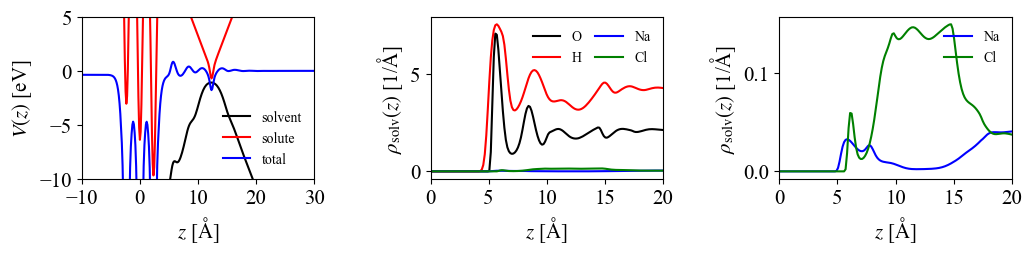### 2-5. ESM-RISM calculation for Al(111) slab with explicit solvent ion (Cl$^-$) in the NaCl aq.¶

&control
calculation  = 'scf'
prefix       = 'Al111.NaCl_aq'
pseudo_dir   = './pseudo'
outdir       = './output'
trism        = .true.
/
&system
ibrav = 0
ntyp = 2, nat = 28
ecutwfc = 40.0, ecutrho = 320.0
occupations = 'smearing', smearing = 'gauss', degauss = 0.01
assume_isolated = "esm", esm_bc = "bc1", tot_charge = -1.0
/
&electrons
electron_maxstep = 200
mixing_beta = 0.1
diagonalization = 'rmm', diago_rmm_conv = .false., diago_rmm_ndim = 4
/
&rism
nsolv    = 3, closure  = 'kh'
tempv    = 300.0  ! Kelvin
ecutsolv = 160.0  ! Rydberg
!
! Lennard-Jones for each atom
!
! For Al using universal force field
solute_lj(1) = 'uff'
! For Aqueous Cl-
solute_lj(2) = 'none'
solute_epsilon(2) = 0.1001
solute_sigma(  2) = 4.4000
!
! 1D-RISM's setting
!
starting1d      = 'zero'
rism1d_conv_thr = 1.0e-8
rism1d_maxstep  = 20000
mdiis1d_size    = 20
mdiis1d_step    = 0.5
!
! 3D-RISM's setting
!
starting3d      = 'zero'
rism3d_maxstep  = 10000
rism3d_conv_thr = 1.0e-6
rism3d_conv_level = 1.0
!
! For Laue-RISM calculation
! in a.u.
!
laue_expand_right = 100.0
/
ATOMIC_SPECIES
Al  -1.0  Al.pbe-nl-rrkjus_psl.1.0.0.UPF
Cl  -1.0  Cl.pbe-nl-rrkjus_psl.1.0.0.UPF

CELL_PARAMETERS angstrom
8.57013418798096 0.00000000000000 0.00000000000000
4.28506709399048 7.42195392063303 0.00000000000000
0.00000000000000 0.00000000000000 35.0000000000000

ATOMIC_POSITIONS angstrom
Al 1.4283556980 0.8246615467 -2.3324950875
Al 4.2850670940 0.8246615467 -2.3324950875
Al 7.1417784900 0.8246615467 -2.3324950875
Al 2.8567113960 3.2986461869 -2.3324950875
Al 5.7134227920 3.2986461869 -2.3324950875
Al 8.5701341880 3.2986461869 -2.3324950875
Al 4.2850670940 5.7726308272 -2.3324950875
Al 7.1417784900 5.7726308272 -2.3324950875
Al 9.9984898860 5.7726308272 -2.3324950875
Al 0.0000000000 1.6493230935 0.0000000000
Al 2.8567113960 1.6493230935 0.0000000000
Al 5.7134227920 1.6493230935 0.0000000000
Al 1.4283556980 4.1233077337 0.0000000000
Al 4.2850670940 4.1233077337 0.0000000000
Al 7.1417784900 4.1233077337 0.0000000000
Al 2.8567113960 6.5972923739 0.0000000000
Al 5.7134227920 6.5972923739 0.0000000000
Al 8.5701341880 6.5972923739 0.0000000000
Al 0.0000000000 0.0000000000 2.3324950875
Al 2.8567113960 0.0000000000 2.3324950875
Al 5.7134227920 0.0000000000 2.3324950875
Al 1.4283556980 2.4739846402 2.3324950875
Al 4.2850670940 2.4739846402 2.3324950875
Al 7.1417784900 2.4739846402 2.3324950875
Al 2.8567113960 4.9479692804 2.3324950875
Al 5.7134227920 4.9479692804 2.3324950875
Al 8.5701341880 4.9479692804 2.3324950875
Cl 6.4276006410 3.7109769603 12.3324950875

K_POINTS {automatic}
2 2 1 1 1 0

SOLVENTS {mol/L}
H2O  -1.0  H2O.spc.MOL
Na+   1.0  Na+.aq.MOL
Cl-   1.0  Cl-.aq.MOL
In :
pt.plot_rism_NaCl_aq1( path = './data/Al111Cl-.NaCl_aq',
prefix = 'Al111.NaCl_aq' )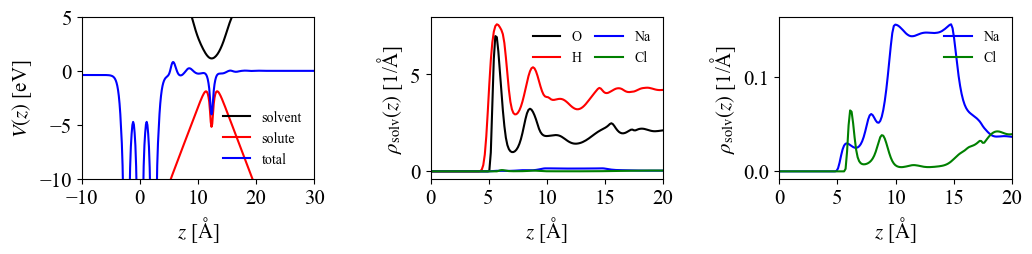# 3. Determining Standard Hydrogen Electrode potential using ESM-RISM calculation¶

## Grand potential calculation¶

We plot grand potential ($\Omega$) profile for left- and right-hand sides of following chemical reaction.

$$1/2 \mathrm{H}_2 (\mathrm{gas}) +\mathrm{H}_2\mathrm{O} (\mathrm{S}) \rightleftharpoons \mathrm{H}_3\mathrm{O}^+ + e^- (\mathrm{M})$$

Definitions of $\Omega$ for left and right ($\Omega_L$ and $\Omega_R$) states:

\begin{align} \Omega_{\mathrm{L}}&=1/2[A(\mathrm{H}_2, \mathrm{gas})+E_{\rm zp}(\mathrm{H}_2)]+\Omega(\mathrm{M}+\mathrm{H}_2\mathrm{O}, \mathrm{S})+E_{\rm zp}(\mathrm{H}_2\mathrm{O}) \\ \Omega_{\mathrm{R}}&=\Omega(\mathrm{M}+\mathrm{H}_3\mathrm{O}^+, \mathrm{S})+E_{\rm zp}(\mathrm{H}_3\mathrm{O}^+) \\ E(\mathrm{H}^+/\mathrm{H}_2)[\mathrm{vs. \ } E^{\rm S}_{\rm vac}]&=-\mu^{\mathrm{SHE}}_{\mathrm{e}} /\mathrm{e} [\mathrm{vs} \Phi_{\rm S}]. \end{align}

Here, M indicates metal electrode. In this notebook, we used Pt(111) surface as the electrode.

## Grand potential profile with and without explicit solvent¶

Here, we calculate the electrode potentials with explicit solvent (H$_2$O or H$_3$O$^+$).
Input files are shown below.

### Input file: Pt(111) clean surface¶

tot_charge was changed from -1.0 to +0.8.

&control
calculation  = 'scf'
prefix       = '0.00'
pseudo_dir   = './pseudo'
outdir       = './data'
trism        = .true.
/
&system
ibrav = 0
ntyp = 1, nat = 64
ecutwfc = 40.0, ecutrho = 320.0
occupations = 'smearing', smearing = 'gauss', degauss = 0.01
assume_isolated = "esm", esm_bc = "bc1", tot_charge = 0.0
/
&electrons
electron_maxstep = 200
mixing_beta = 0.1
diagonalization = 'rmm', diago_rmm_conv = .false., diago_rmm_ndim = 4
/
&rism
nsolv    = 3
closure  = 'kh'
tempv    = 300.0  ! Kelvin
ecutsolv = 160.0  ! Rydberg
!
! Lennard-Jones for each atom
!
! For Pt using fitted LJ-potential
!
solute_lj(1) = 'none',solute_epsilon(1) = 1.66, solute_sigma(1)=2.65
!
! 1D-RISM's setting
!
starting1d      = 'zero'
rism1d_conv_thr = 1.0e-8
rism1d_maxstep  = 100000
mdiis1d_size    = 20
mdiis1d_step    = 0.1
!
! 3D-RISM's setting
!
starting3d      = 'zero'
rism3d_maxstep  = 10000
rism3d_conv_thr = 1.0e-6
rism3d_conv_level = 0.5
mdiis3d_size    = 20
mdiis3d_step    = 0.8
!
! For Laue-RISM calculation
! in a.u.
!
laue_expand_right = 60.0
laue_starting_right = -8.20
laue_buffer_right = 10.0
/
ATOMIC_SPECIES
Pt  -1.0  Pt.pbe-n-van.UPF

CELL_PARAMETERS angstrom
11.08743432900506 0.00000000000000 0.00000000000000
5.54371716450253 9.60199979171006 0.00000000000000
0.00000000000000 0.00000000000000 40.000

ATOMIC_POSITIONS angstrom
Pt 0.0000000000 0.0000000000 -10.0000000000
Pt 2.7718585823 0.0000000000 -10.0000000000
Pt 5.5437171645 0.0000000000 -10.0000000000
Pt 8.3155757468 0.0000000000 -10.0000000000
Pt 1.3859292911 2.4004999479 -10.0000000000
Pt 4.1577878734 2.4004999479 -10.0000000000
Pt 6.9296464556 2.4004999479 -10.0000000000
Pt 9.7015050379 2.4004999479 -10.0000000000
Pt 2.7718585823 4.8009998959 -10.0000000000
Pt 5.5437171645 4.8009998959 -10.0000000000
Pt 8.3155757468 4.8009998959 -10.0000000000
Pt 11.0874343290 4.8009998959 -10.00000000000
Pt 4.1577878734 7.2014998438 -10.0000000000
Pt 6.9296464556 7.2014998438 -10.0000000000
Pt 9.7015050379 7.2014998438 -10.0000000000
Pt 12.4733636201 7.2014998438 -10.00000000000
Pt 1.3859292911 0.8001666493 -7.7367869448
Pt 4.1577878734 0.8001666493 -7.7367869448
Pt 6.9296464556 0.8001666493 -7.7367869448
Pt 9.7015050379 0.8001666493 -7.7367869448
Pt 2.7718585823 3.2006665972 -7.7367869448
Pt 5.5437171645 3.2006665972 -7.7367869448
Pt 8.3155757468 3.2006665972 -7.7367869448
Pt 11.0874343290 3.2006665972 -7.7367869448
Pt 4.1577878734 5.6011665452 -7.7367869448
Pt 6.9296464556 5.6011665452 -7.7367869448
Pt 9.7015050379 5.6011665452 -7.7367869448
Pt 12.4733636201 5.6011665452 -7.7367869448
Pt 5.5437171645 8.0016664931 -7.7367869448
Pt 8.3155757468 8.0016664931 -7.7367869448
Pt 11.0874343290 8.0016664931 -7.7367869448
Pt 13.8592929113 8.0016664931 -7.7367869448
Pt -0.0000000000 1.6003332986 -5.4735738896
Pt 2.7718585823 1.6003332986 -5.4735738896
Pt 5.5437171645 1.6003332986 -5.4735738896
Pt 8.3155757468 1.6003332986 -5.4735738896
Pt 1.3859292911 4.0008332465 -5.4735738896
Pt 4.1577878734 4.0008332465 -5.4735738896
Pt 6.9296464556 4.0008332465 -5.4735738896
Pt 9.7015050379 4.0008332465 -5.4735738896
Pt 2.7718585823 6.4013331945 -5.4735738896
Pt 5.5437171645 6.4013331945 -5.4735738896
Pt 8.3155757468 6.4013331945 -5.4735738896
Pt 11.0874343290 6.4013331945 -5.4735738896
Pt 4.1577878734 8.8018331424 -5.4735738896
Pt 6.9296464556 8.8018331424 -5.4735738896
Pt 9.7015050379 8.8018331424 -5.4735738896
Pt 12.4733636201 8.8018331424 -5.4735738896
Pt 0.0000000000 0.0000000000 -3.2103608344
Pt 2.7718585823 0.0000000000 -3.2103608344
Pt 5.5437171645 0.0000000000 -3.2103608344
Pt 8.3155757468 0.0000000000 -3.2103608344
Pt 1.3859292911 2.4004999479 -3.2103608344
Pt 4.1577878734 2.4004999479 -3.2103608344
Pt 6.9296464556 2.4004999479 -3.2103608344
Pt 9.7015050379 2.4004999479 -3.2103608344
Pt 2.7718585823 4.8009998959 -3.2103608344
Pt 5.5437171645 4.8009998959 -3.2103608344
Pt 8.3155757468 4.8009998959 -3.2103608344
Pt 11.0874343290 4.8009998959 -3.2103608344
Pt 4.1577878734 7.2014998438 -3.2103608344
Pt 6.9296464556 7.2014998438 -3.2103608344
Pt 9.7015050379 7.2014998438 -3.2103608344
Pt 12.4733636201 7.2014998438 -3.2103608344

K_POINTS {automatic}
2 2 1 1 1 0

SOLVENTS {mol/L}
H2O  -1.0  H2O.tip5p.MOL
H3O+  1.0  H3O+.chuev.MOL
Cl-   1.0  Cl-.aq.MOL

### Input file: Pt(111) with H$_2$O molecule¶

tot_charge was changed from -1.0 to 0.0.

&control
calculation  = 'scf'
prefix       = '0.00'
pseudo_dir   = './pseudo'
outdir       = './data'
trism        = .true.
/
&system
ibrav = 0
ntyp = 3, nat = 67
ecutwfc = 40.0, ecutrho = 320.0
occupations = 'smearing', smearing = 'gauss', degauss = 0.01
assume_isolated = "esm", esm_bc = "bc1", tot_charge = 0.0
/
&electrons
electron_maxstep = 200
mixing_beta = 0.1
diagonalization = 'rmm', diago_rmm_conv = .false., diago_rmm_ndim = 4
/
&rism
nsolv    = 3
closure  = 'kh'
tempv    = 300.0  ! Kelvin
ecutsolv = 160.0  ! Rydberg
!
! Lennard-Jones for each atom
!
! For Pt using fitted LJ-potential
!
solute_lj(1) = 'none',solute_epsilon(1) = 1.66, solute_sigma(1)=2.65
!
! For H
!
solute_lj(2) = 'none'
solute_epsilon(2) = 0.046
solute_sigma(  2) = 1.000
!
! For O
!
solute_lj(3) = 'none'
solute_epsilon(3) = 0.1600
solute_sigma(  3) = 3.1200
!
! 1D-RISM's setting
!
starting1d      = 'zero'
rism1d_conv_thr = 1.0e-8
rism1d_maxstep  = 100000
mdiis1d_size    = 20
mdiis1d_step    = 0.1
!
! 3D-RISM's setting
!
starting3d      = 'zero'
rism3d_maxstep  = 10000
rism3d_conv_thr = 1.0e-6
rism3d_conv_level = 0.5
mdiis3d_size    = 20
mdiis3d_step    = 0.8
!
! For Laue-RISM calculation
! in a.u.
!
laue_expand_right = 60.0
laue_starting_right = -8.20
laue_buffer_right = 10.0
/
ATOMIC_SPECIES
Pt  -1.0  Pt.pbe-n-van.UPF
H   -1.0  H.pbe-rrkjus_psl.0.1.UPF
O   -1.0  O.pbe-rrkjus.UPF

CELL_PARAMETERS angstrom
11.08743432900506 0.00000000000000 0.00000000000000
5.54371716450253 9.60199979171006 0.00000000000000
0.00000000000000 0.00000000000000 40.000

ATOMIC_POSITIONS angstrom
Pt 0.0000000000 0.0000000000 -10.0000000000  0 0 0
Pt 2.7718585823 0.0000000000 -10.0000000000  0 0 0
Pt 5.5437171645 0.0000000000 -10.0000000000  0 0 0
Pt 8.3155757468 0.0000000000 -10.0000000000  0 0 0
Pt 1.3859292911 2.4004999479 -10.0000000000  0 0 0
Pt 4.1577878734 2.4004999479 -10.0000000000  0 0 0
Pt 6.9296464556 2.4004999479 -10.0000000000  0 0 0
Pt 9.7015050379 2.4004999479 -10.0000000000  0 0 0
Pt 2.7718585823 4.8009998959 -10.0000000000  0 0 0
Pt 5.5437171645 4.8009998959 -10.0000000000  0 0 0
Pt 8.3155757468 4.8009998959 -10.0000000000  0 0 0
Pt 11.0874343290 4.8009998959 -10.0000000000  0 0 0
Pt 4.1577878734 7.2014998438 -10.0000000000  0 0 0
Pt 6.9296464556 7.2014998438 -10.0000000000  0 0 0
Pt 9.7015050379 7.2014998438 -10.0000000000  0 0 0
Pt 12.4733636201 7.2014998438 -10.0000000000  0 0 0
Pt 1.3859292911 0.8001666493 -7.7367869448  0 0 0
Pt 4.1577878734 0.8001666493 -7.7367869448  0 0 0
Pt 6.9296464556 0.8001666493 -7.7367869448  0 0 0
Pt 9.7015050379 0.8001666493 -7.7367869448  0 0 0
Pt 2.7718585823 3.2006665972 -7.7367869448  0 0 0
Pt 5.5437171645 3.2006665972 -7.7367869448  0 0 0
Pt 8.3155757468 3.2006665972 -7.7367869448  0 0 0
Pt 11.0874343290 3.2006665972 -7.7367869448  0 0 0
Pt 4.1577878734 5.6011665452 -7.7367869448  0 0 0
Pt 6.9296464556 5.6011665452 -7.7367869448  0 0 0
Pt 9.7015050379 5.6011665452 -7.7367869448  0 0 0
Pt 12.4733636201 5.6011665452 -7.7367869448  0 0 0
Pt 5.5437171645 8.0016664931 -7.7367869448  0 0 0
Pt 8.3155757468 8.0016664931 -7.7367869448  0 0 0
Pt 11.0874343290 8.0016664931 -7.7367869448  0 0 0
Pt 13.8592929113 8.0016664931 -7.7367869448  0 0 0
Pt -0.0000000000 1.6003332986 -5.4735738896  0 0 0
Pt 2.7718585823 1.6003332986 -5.4735738896  0 0 0
Pt 5.5437171645 1.6003332986 -5.4735738896  0 0 0
Pt 8.3155757468 1.6003332986 -5.4735738896  0 0 0
Pt 1.3859292911 4.0008332465 -5.4735738896  0 0 0
Pt 4.1577878734 4.0008332465 -5.4735738896  0 0 0
Pt 6.9296464556 4.0008332465 -5.4735738896  0 0 0
Pt 9.7015050379 4.0008332465 -5.4735738896  0 0 0
Pt 2.7718585823 6.4013331945 -5.4735738896  0 0 0
Pt 5.5437171645 6.4013331945 -5.4735738896  0 0 0
Pt 8.3155757468 6.4013331945 -5.4735738896  0 0 0
Pt 11.0874343290 6.4013331945 -5.4735738896  0 0 0
Pt 4.1577878734 8.8018331424 -5.4735738896  0 0 0
Pt 6.9296464556 8.8018331424 -5.4735738896  0 0 0
Pt 9.7015050379 8.8018331424 -5.4735738896  0 0 0
Pt 12.4733636201 8.8018331424 -5.4735738896  0 0 0
Pt 0.0000000000 0.0000000000 -3.2103608344  0 0 0
Pt 2.7718585823 0.0000000000 -3.2103608344  0 0 0
Pt 5.5437171645 0.0000000000 -3.2103608344  0 0 0
Pt 8.3155757468 0.0000000000 -3.2103608344  0 0 0
Pt 1.3859292911 2.4004999479 -3.2103608344  0 0 0
Pt 4.1577878734 2.4004999479 -3.2103608344  0 0 0
Pt 6.9296464556 2.4004999479 -3.2103608344  0 0 0
Pt 9.7015050379 2.4004999479 -3.2103608344  0 0 0
Pt 2.7718585823 4.8009998959 -3.2103608344  0 0 0
Pt 5.5437171645 4.8009998959 -3.2103608344  0 0 0
Pt 8.3155757468 4.8009998959 -3.2103608344  0 0 0
Pt 11.0874343290 4.8009998959 -3.2103608344  0 0 0
Pt 4.1577878734 7.2014998438 -3.2103608344  0 0 0
Pt 6.9296464556 7.2014998438 -3.2103608344  0 0 0
Pt 9.7015050379 7.2014998438 -3.2103608344  0 0 0
Pt 12.4733636201 7.2014998438 -3.2103608344  0 0 0
H 8.3155757468 4.8009998959 6.7896391656
H 6.8046757468 4.8009998959 6.7896391656
O 7.5601257468 5.3899498959 6.7896391656

K_POINTS {automatic}
2 2 1 1 1 0

SOLVENTS {mol/L}
H2O  -1.0  H2O.tip5p.MOL
H3O+  1.0  H3O+.chuev.MOL
Cl-   1.0  Cl-.aq.MOL

### Input file: Pt(111) with H$_3$O$^+$ ion¶

tot_charge was changed from 0.0 to 1.0.

&control
calculation  = 'scf'
prefix       = '0.00'
pseudo_dir   = './pseudo'
outdir       = './data'
trism        = .true.
/
&system
ibrav = 0
ntyp = 3, nat = 68
ecutwfc = 40.0, ecutrho = 320.0
occupations = 'smearing', smearing = 'gauss', degauss = 0.01
assume_isolated = "esm", esm_bc = "bc1", tot_charge = 1.0
starting_charge(2)=0.33
/
&electrons
electron_maxstep = 200
mixing_beta = 0.1
diagonalization = 'rmm', diago_rmm_conv = .false., diago_rmm_ndim = 4
/
&rism
nsolv    = 3
closure  = 'kh'
tempv    = 300.0  ! Kelvin
ecutsolv = 160.0  ! Rydberg
!
! Lennard-Jones for each atom
!
! For Pt using fitted LJ-potential
!
solute_lj(1) = 'none',solute_epsilon(1) = 1.66, solute_sigma(1)=2.65
!
! For H
!
solute_lj(2) = 'none'
solute_epsilon(2) = 0.046
solute_sigma(  2) = 1.000
!
! For O
!
solute_lj(3) = 'none'
solute_epsilon(3) = 0.1600
solute_sigma(  3) = 3.1200
!
! 1D-RISM's setting
!
starting1d      = 'zero'
rism1d_conv_thr = 1.0e-8
rism1d_maxstep  = 100000
mdiis1d_size    = 20
mdiis1d_step    = 0.1
!
! 3D-RISM's setting
!
starting3d      = 'zero'
rism3d_maxstep  = 10000
rism3d_conv_thr = 1.0e-6
rism3d_conv_level = 0.5
mdiis3d_size    = 20
mdiis3d_step    = 0.8
!
! For Laue-RISM calculation
! in a.u.
!
laue_expand_right = 60.0
laue_starting_right = -8.20
laue_buffer_right = 10.0
/
ATOMIC_SPECIES
Pt  -1.0  Pt.pbe-n-van.UPF
H   -1.0  H.pbe-rrkjus_psl.0.1.UPF
O   -1.0  O.pbe-rrkjus.UPF

CELL_PARAMETERS angstrom
11.08743432900506 0.00000000000000 0.00000000000000
5.54371716450253 9.60199979171006 0.00000000000000
0.00000000000000 0.00000000000000 40.000

ATOMIC_POSITIONS angstrom
Pt 0.0000000000 0.0000000000 -10.0000000000  0 0 0
Pt 2.7718585823 0.0000000000 -10.0000000000  0 0 0
Pt 5.5437171645 0.0000000000 -10.0000000000  0 0 0
Pt 8.3155757468 0.0000000000 -10.0000000000  0 0 0
Pt 1.3859292911 2.4004999479 -10.0000000000  0 0 0
Pt 4.1577878734 2.4004999479 -10.0000000000  0 0 0
Pt 6.9296464556 2.4004999479 -10.0000000000  0 0 0
Pt 9.7015050379 2.4004999479 -10.0000000000  0 0 0
Pt 2.7718585823 4.8009998959 -10.0000000000  0 0 0
Pt 5.5437171645 4.8009998959 -10.0000000000  0 0 0
Pt 8.3155757468 4.8009998959 -10.0000000000  0 0 0
Pt 11.0874343290 4.8009998959 -10.0000000000  0 0 0
Pt 4.1577878734 7.2014998438 -10.0000000000  0 0 0
Pt 6.9296464556 7.2014998438 -10.0000000000  0 0 0
Pt 9.7015050379 7.2014998438 -10.0000000000  0 0 0
Pt 12.4733636201 7.2014998438 -10.0000000000  0 0 0
Pt 1.3859292911 0.8001666493 -7.7367869448  0 0 0
Pt 4.1577878734 0.8001666493 -7.7367869448  0 0 0
Pt 6.9296464556 0.8001666493 -7.7367869448  0 0 0
Pt 9.7015050379 0.8001666493 -7.7367869448  0 0 0
Pt 2.7718585823 3.2006665972 -7.7367869448  0 0 0
Pt 5.5437171645 3.2006665972 -7.7367869448  0 0 0
Pt 8.3155757468 3.2006665972 -7.7367869448  0 0 0
Pt 11.0874343290 3.2006665972 -7.7367869448  0 0 0
Pt 4.1577878734 5.6011665452 -7.7367869448  0 0 0
Pt 6.9296464556 5.6011665452 -7.7367869448  0 0 0
Pt 9.7015050379 5.6011665452 -7.7367869448  0 0 0
Pt 12.4733636201 5.6011665452 -7.7367869448  0 0 0
Pt 5.5437171645 8.0016664931 -7.7367869448  0 0 0
Pt 8.3155757468 8.0016664931 -7.7367869448  0 0 0
Pt 11.0874343290 8.0016664931 -7.7367869448  0 0 0
Pt 13.8592929113 8.0016664931 -7.7367869448  0 0 0
Pt -0.0000000000 1.6003332986 -5.4735738896  0 0 0
Pt 2.7718585823 1.6003332986 -5.4735738896  0 0 0
Pt 5.5437171645 1.6003332986 -5.4735738896  0 0 0
Pt 8.3155757468 1.6003332986 -5.4735738896  0 0 0
Pt 1.3859292911 4.0008332465 -5.4735738896  0 0 0
Pt 4.1577878734 4.0008332465 -5.4735738896  0 0 0
Pt 6.9296464556 4.0008332465 -5.4735738896  0 0 0
Pt 9.7015050379 4.0008332465 -5.4735738896  0 0 0
Pt 2.7718585823 6.4013331945 -5.4735738896  0 0 0
Pt 5.5437171645 6.4013331945 -5.4735738896  0 0 0
Pt 8.3155757468 6.4013331945 -5.4735738896  0 0 0
Pt 11.0874343290 6.4013331945 -5.4735738896  0 0 0
Pt 4.1577878734 8.8018331424 -5.4735738896  0 0 0
Pt 6.9296464556 8.8018331424 -5.4735738896  0 0 0
Pt 9.7015050379 8.8018331424 -5.4735738896  0 0 0
Pt 12.4733636201 8.8018331424 -5.4735738896  0 0 0
Pt 0.0000000000 0.0000000000 -3.2103608344  0 0 0
Pt 2.7718585823 0.0000000000 -3.2103608344  0 0 0
Pt 5.5437171645 0.0000000000 -3.2103608344  0 0 0
Pt 8.3155757468 0.0000000000 -3.2103608344  0 0 0
Pt 1.3859292911 2.4004999479 -3.2103608344  0 0 0
Pt 4.1577878734 2.4004999479 -3.2103608344  0 0 0
Pt 6.9296464556 2.4004999479 -3.2103608344  0 0 0
Pt 9.7015050379 2.4004999479 -3.2103608344  0 0 0
Pt 2.7718585823 4.8009998959 -3.2103608344  0 0 0
Pt 5.5437171645 4.8009998959 -3.2103608344  0 0 0
Pt 8.3155757468 4.8009998959 -3.2103608344  0 0 0
Pt 11.0874343290 4.8009998959 -3.2103608344  0 0 0
Pt 4.1577878734 7.2014998438 -3.2103608344  0 0 0
Pt 6.9296464556 7.2014998438 -3.2103608344  0 0 0
Pt 9.7015050379 7.2014998438 -3.2103608344  0 0 0
Pt 12.4733636201 7.2014998438 -3.2103608344  0 0 0
H 8.6355757468 5.7429998959 6.7896391656
H 8.6355757468 4.3299998959 7.6056391656
H 8.6355757468 4.3299998959 5.9736391656
O 8.3155757468 4.8009998959 6.7896391656  0 0 0

K_POINTS {automatic}
2 2 1 1 1 0

SOLVENTS {mol/L}
H2O  -1.0  H2O.tip5p.MOL
H3O+  1.0  H3O+.chuev.MOL
Cl-   1.0  Cl-.aq.MOL

## Result of Grand potential profiles¶

In :
file1='./data/SHE/Pt(111).dat'
file2='./data/SHE/Pt_energy.dat'
pt.Omega(file1, file2)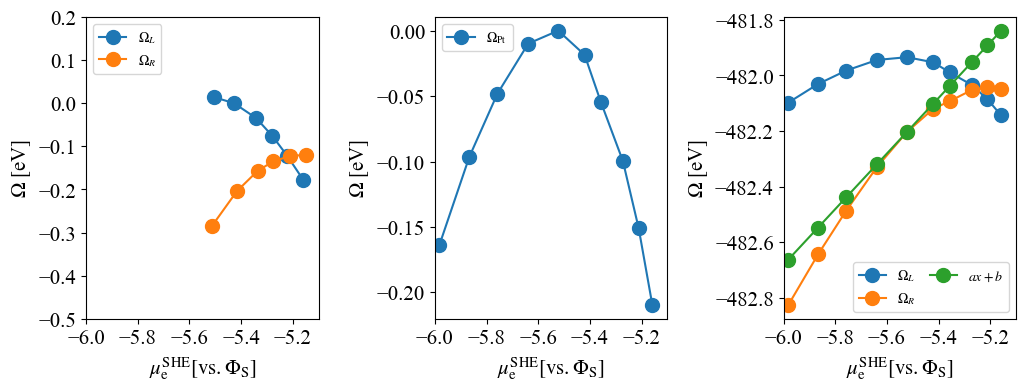Left: $\Omega(\mu)$ with explicit solvent
Right: $\Omega(\mu)$ without explicit solvent

Intersection points for left and right panels are $\mu=-5.23$eV and $\mu=-5.26$eV, respectively.

# SHE potential without electrode¶

SHE potential can be also calculated by Helmholtz's free energy difference:

\begin{align} G_{\mathrm{L}}&=1/2[A(\mathrm{H}_2, \mathrm{gas})+E_{\rm zp}(\mathrm{H}_2)]+A(\mathrm{H}_2\mathrm{O}, \mathrm{S})+E_{\rm zp}(\mathrm{H}_2\mathrm{O}) \\ G_{\mathrm{R}}&=A(\mathrm{H}_3\mathrm{O}^+, \mathrm{S})+E_{\rm zp}(\mathrm{H}_3\mathrm{O}^+) \end{align}

This result is shown as following:

In :
Ry2eV=27.2116*0.5
TS_H2=-0.404
A_H2=-2.3322957476*Ry2eV+TS_H2
A_H2O=-34.2914608381*Ry2eV
A_H3O=-35.1019053889*Ry2eV

Ezp_H2=0.270
Ezp_H2O=0.562
Ezp_H3O=0.909

G_L=0.5*(A_H2+Ezp_H2)+A_H2O+Ezp_H2O
G_R=A_H3O+Ezp_H3O

print('E(H+/H2)[vs. E_vac] (eV) = '+str(-(G_L-G_R)))

E(H+/H2)[vs. E_vac] (eV) = 5.2536282720733425


This value (E(H+/H2)[vs. E_vac]=5.25eV) is almost agree with the intersection points obtained by the grand potential profiles.
Input files are also shown below.

## Input file: H$_2$O molecule¶

&CONTROL
calculation  = 'relax'       ! 'scf' 'relax'
outdir       = './data'
pseudo_dir   = './pseudo'
prefix       = 'H2O.HCl_aq'
trism        = .true.
!  lfcp         = .true.
max_seconds  =  72000
nstep        = 100
/
&SYSTEM
ibrav = 1, A = 20.0
ntyp = 2, nat = 3
nbnd = 20
ecutwfc = 40, ecutrho = 320
occupations = "smearing", smearing = "gauss", degauss = 0.01
assume_isolated = "esm", esm_bc = "bc1", tot_charge = 0.0
!  starting_charge(2) = 0.33
/
&ELECTRONS
electron_maxstep = 500, mixing_beta = 0.1, !conv_thr = 1.0d-8
diagonalization = 'rmm', diago_rmm_conv = .false., diago_rmm_ndim = 4
!  startingwfc = 'file'
!  startingpot = 'file'
/
&IONS
ion_dynamics = 'bfgs'
!  upscale = 1.d0
/
&RISM
nsolv = 3, closure  = 'kh'
tempv = 300, ecutsolv = 160
!Lennard-Jones
solute_lj(1) = 'none', solute_epsilon(1) = 0.1554, solute_sigma(1) = 3.166 !TIP5P O
solute_lj(2) = 'none', solute_epsilon(2) = 0.0460, solute_sigma(2) = 1.000 !modified H
!Laue-RISM
laue_expand_left = 60
laue_expand_right = 60
rism3d_maxstep = 10000
rism3d_conv_thr = 1.0d-6
rism3d_conv_level = 0.5
!  starting1d = "file"
!  starting3d = "file"
/

K_POINTS gamma

SOLVENTS {mol/L}
H2O  -1.0  H2O.tip5p.MOL
H3O+  1.0  H3O+.chuev.MOL
Cl-   1.0  Cl-.aq.MOL

ATOMIC_SPECIES
O   16.00  O.pbe-rrkjus.UPF
H   1.008  H.pbe-rrkjus_psl.0.1.UPF

ATOMIC_POSITIONS {angstrom}
O        0.   0.   0.
H        0.6  0.6  0.6
H        0.6  0.6 -0.6

## Input file: H$_3$O$^+$ ion¶

&CONTROL
calculation  = 'relax'       ! 'scf' 'relax'
outdir       = './data'
pseudo_dir   = './pseudo'
prefix       = 'H2O.HCl_aq'
trism        = .true.
!  lfcp         = .true.
max_seconds  =  72000
nstep        = 100
/
&SYSTEM
ibrav = 1, A = 20.0
ntyp = 2, nat = 4
nbnd = 20
ecutwfc = 40, ecutrho = 320
occupations = "smearing", smearing = "gauss", degauss = 0.01
assume_isolated = "esm", esm_bc = "bc1", tot_charge = 1.0
!  starting_charge(2) = 0.33
/
&ELECTRONS
electron_maxstep = 500, mixing_beta = 0.1, !conv_thr = 1.0d-8
diagonalization = 'rmm', diago_rmm_conv = .false., diago_rmm_ndim = 4
!  startingwfc = 'file'
!  startingpot = 'file'
/
&IONS
ion_dynamics = 'bfgs'
!  upscale = 1.d0
/
&RISM
nsolv = 3, closure  = 'kh'
tempv = 300, ecutsolv = 160
!Lennard-Jones
solute_lj(1) = 'none', solute_epsilon(1) = 0.1554, solute_sigma(1) = 3.166 !TIP5P O
solute_lj(2) = 'none', solute_epsilon(2) = 0.0460, solute_sigma(2) = 1.000 !modified H
!Laue-RISM
laue_expand_left = 60
laue_expand_right = 60
rism3d_maxstep = 10000
rism3d_conv_thr = 1.0d-6
rism3d_conv_level = 0.5
!  starting1d = "file"
!  starting3d = "file"
/

K_POINTS gamma

SOLVENTS {mol/L}
H2O  -1.0  H2O.tip5p.MOL
H3O+  1.0  H3O+.chuev.MOL
Cl-   1.0  Cl-.aq.MOL

ATOMIC_SPECIES
O   16.00  O.pbe-rrkjus.UPF
H   1.008  H.pbe-rrkjus_psl.0.1.UPF

ATOMIC_POSITIONS {angstrom}
O        0.   0.   0.
H        0.6  0.6  0.6
H        0.6  0.6 -0.6
H       -0.6 -0.5  0.